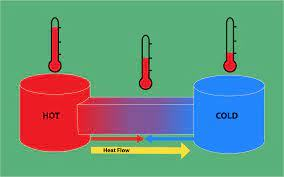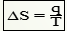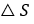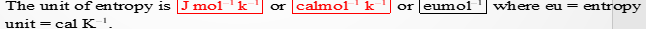# Chemical ThermodynamicsIntroduction

There are so many natural phenomena which occur along a particular direction. To answer the feasibility of a process along a particular direction, second law of thermodynamics is required. Before discussing about the second law of thermodynamics, we shall discuss about spontaneous process and criteria for spontaneity.

Spontaneous process and non-spontaneous process

The processes which proceed by themselves without any external additional effort are called spontaneous processes. All the natural process are spontaneous process.

The processes which can’t proceed by themselves are called non spontaneous process. Such process can’t proceed without any external conflict.

Entropy

Entropy is the measurement of randomness or disorderness of the molecule in the system. It is denoted by “S”. More is the disorderness of the molecule in the system., higher is the value of entropy. For example, if we heat any object, the velocity of the molecule increases. It means entropy of the substance also increases.

If the system absorbs a q amount of heat at temperature “T” then increase in entropy of the systemIf system absorb q amount of heat, then q will be positive and entropy change also be positive. Positiveindicates the entropy of the system increases. If system lose q amount of heat, then q will be negative quantity. Negativeindicates that entropy of the system decreases.Second law of thermodynamics:

Statement:

For any spontaneous process, the entropy change of the universe is always positive i.e.Explanation

Melting of ice at room temperature

In this process q amount of heat reversibly from surrounding. So, entropy change of the ice isFreezing of water at 0ºC

In this process water lose q amount of heat reversibly to surrounding. So entropy change of water (system)